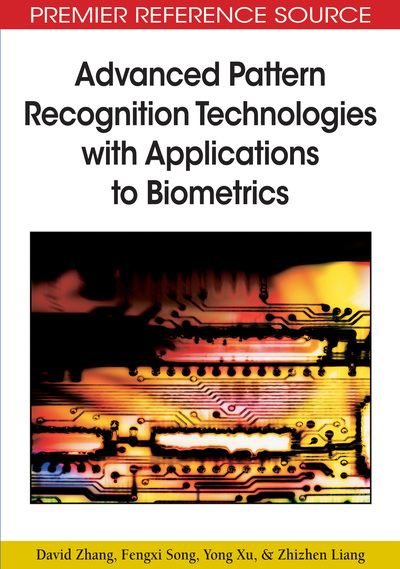# Other Tensor Analysis and Further Direction

David Zhang (Hong Kong Polytechnic University, Hong Kong), Fengxi Song (New Star Research Institute Of Applied Technology, China), Yong Xu (Harbin Institute of Technology, China) and Zhizhen Liang (Shanghai Jiao Tong University, China)
DOI: 10.4018/978-1-60566-200-8.ch011

## Abstract

In this chapter, we describe tensor-based classifiers, tensor canonical correlation analysis and tensor partial least squares, which can be used in biometrics. Section 11.1 gives background and devolvement of these tensor methods. Section 11.2 introduces tensor-based classifiers. Section 11.3 gives tensor canonical correlation analysis and tensor partial least squares. We summarize this chapter in Section 11.4.
Chapter Preview
Top

## Introduction

In general, a biometric system consists of data acquisition phase, feature extraction phase and the classification phase. In the data acquisition phase, the data obtained are often represented by multidimensional arrays, that is, tensors, such as the grey face image, the colour faces in image classification and the gene expression data. In the feature extraction phase, multilinear subspace methods mentioned in Chapters 8, 9, and 10 can be used for data representation and feature extraction. In the classification phase, the classifiers (Bousquet, Boucheron, & Lugosi, 2004; Duda, Hart, & Stock, 2001; Muller, Mika, Ratsch, Tsuda, & Scholkopf, 2001; Highleyman, 1962) play an important role in the biometric system and how to design a good classifier is of interest for researchers. Traditionally, the classifier design is almost based on vector pattern, that is, before using them, any non-vector pattern such as an image should be first vectorized into the vector pattern by the techniques such as concatenation. However, the Ugly Duckling Theorem (Chen, Wang, & Tian, 2007) indicates that it cannot be said that one pattern representation is always better than another. As a result, it is not always reasonable to design classifiers based on traditional vector patterns.

Motivated by the tensor ideas in feature extraction, some researchers have designed some classifiers based on tensor ideas in recent years. For example, Chen,Wang and Tian (2007) designed the classifiers using a set of given matrix patterns. They first represented a pattern in matrix form and extended existing vector-based classifiers to their corresponding matrixized versions. Specifically, considering a similar principle to the support vector machine which maximizes the separation margin and has superior generalization performance, the modified HK algorithm (MHKS) (Leski, 2003) is chosen and then a matrix-based MHKS (MatMHKS) classifier is developed. Their experimental results on ORL, Letters and UCI data sets show that MatMHKS is more powerful in generalization than MHKS. Further, Wang and Chen (2007) proposed a new classifier based on matrix patterns and LS-SVM. This method is referred to as MatLSSVM. The MatLSSVM method can not only directly operate on original matrix patterns, but also efficiently reduce memory for the weight vector in LS-SVM. However, one of disadvantages of MatLSSVM is that there exist unclassifiable regions when it is extended to the multi-class problems. To avoid this point, a corresponding fuzzy version of MatLSSVM (MatFLSSVM) is further proposed to remove unclassifiable regions for multi-class problems. Experimental results on some benchmark datasets show that their proposed method is competitive in classification performance compared to LS-SVM and fuzzy LS-SVM (FLS-SVM). In Tao, Li, Hu, Maybank and Wu (2005; Tao, Li, Wu, Hu, & Maybank, 2006) a supervised tensor learning (STL) framework is established for convex optimization techniques such as support vector machines (SVM) and minimax probability machines (MPM). Within the STL framework, many conventional learning machines can be generalized to take nth-order tensors as inputs. These generalized algorithms have several advantages: (1) reduce the problem of “the curse of dimensionality” in machine learning and data mining; (2) avoid the failure to converge; and (3) achieve better separation between the different categories of samples. Further, they generalized MPM to the STL version, which is called tensor MPM (TMPM). The TMPM method can obtain a series of tensor projection vector by an iterative algorithm. The experiments on a binary classification problem show that TMPM significantly outperforms the original MPM.

## Complete Chapter List

Search this Book:
Reset